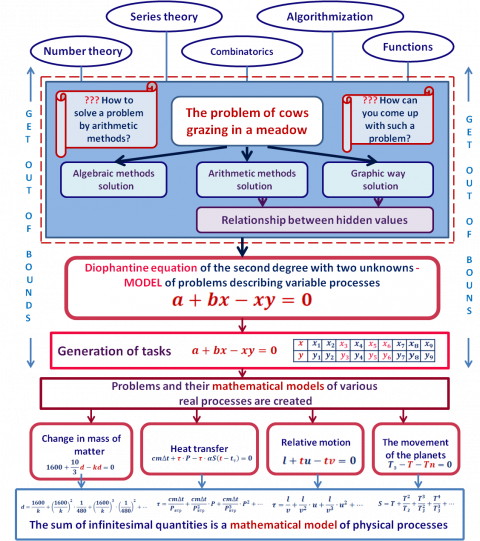# MATHEMATICAL MODELS FOR CREATING PROBLEMS ABOUT REAL PHYSICAL PROCESSESAuthor: ANDRIY SHUT

Menthors: LYUDMILA BEZPERSTOVA, YURIY GULIY

School: UNESCO Centre “Junior Academy of Science of Ukraine” (JASU)

The research work shows the way to create problems that describe similar real processes. An analysis of the solution of one well-known arithmetic problem about cows in a meadow created a mathematical model for generating problems. This is a Diophantine equation of the second degree with two unknowns. The number of solutions of the equation depends on the values of its parameters. If the equation has at least three solutions, we can formulate the problem. It was shown that completely different processes by nature (change of mass, relative motion of a body, change of temperature, motion of celestial bodies) can be described by the same mathematical dependence – a number of infinitesimal quantities. This is another mathematical model. The Diophantine equation of the second degree with two variables is a model that characterizes the state of the process. It is used to generate mathematical and physical problems. Thanks to the ability to change the numerical data, you can get as many new tasks as you like. This is the innovative idea of the study.